## Vortex motion in a quasi one-dimensional Josephson junction array

This page shows solutions of the classical dynamics of the Josephson junction array shown in figure 1. The parameters are chosen in the flux flow regime.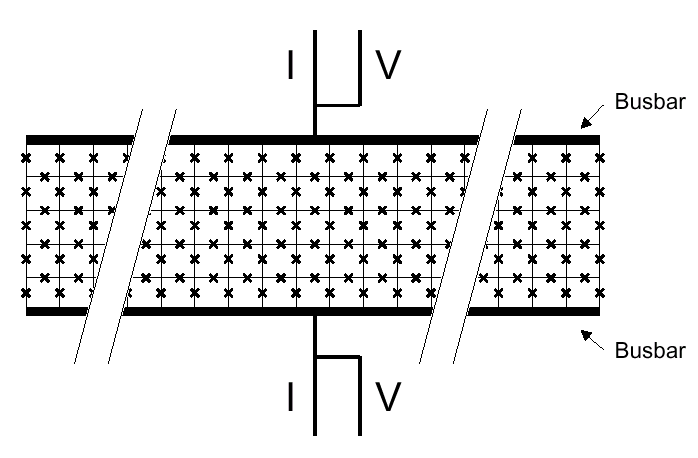Figure 1: Schematic lay out of a Josephson junction array for one-dimensional vortex motion. Josephson junctions are represented by a cross, cells are areas enclosed by four junctions. The arrays simulted have a length of 29 cells and a width of 5 cells between solid superconducting electrodes (busbars).  Vortices are induced by an applied magnetic field perpendicular to the array. They are repelled by the busbars and forced to the middle row. Current is applied vertically in the figure between the busbars, homogeneously along the length. The current induces a potential gradient along the length. The motion of vortices through the array creates a voltage across the array.

The Gauge chosen here is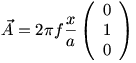.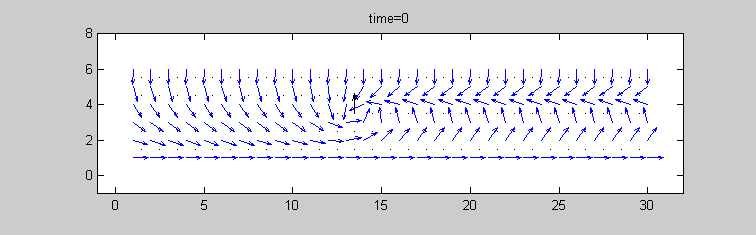Figure 2 a: Phase configuration for 1.5 flux quanta applied to the total array . The Phases of the islands are plotted with blue arrows. The black arrow indicates the position of the vortex according to Eq. (1.4).  The parameters are f=1.5/(5*29),bc=1 and I=15 I0.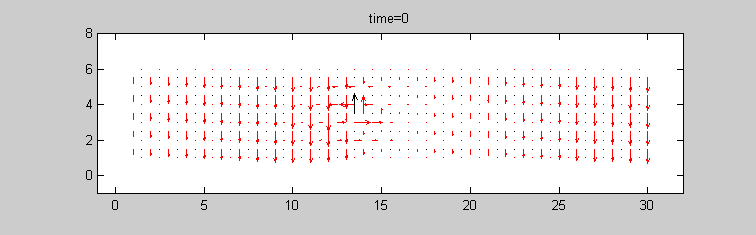Figure 2 b: Current distribution for 1.5 flux quanta applied to the total array. The Phases of the islands are plotted with blue arrows. The black arrow indicates the position of the vortex according to Eq. (1.4). The parameters are f=1.5/(5*29),bc=1 and I=15 I0.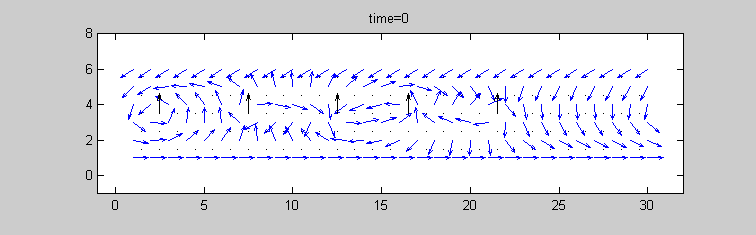Figure 3 a: Phase configuration for six flux quanta applied to the total array. The Phases of the islands are plotted with blue arrows. The black arrow indicates the position of the vortex according to Eq. (1.4). The parameters are f=6/(5*29),bc=1 and I=10 I0.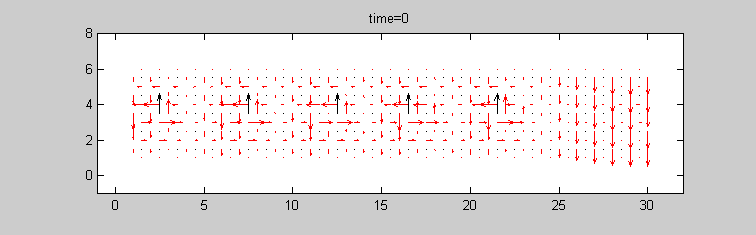Figure 3 b: Current distribution for six flux quanta applied to the total array. The Phases of the islands are plotted with blue arrows. The black arrow indicates the position of the vortex according to Eq. (1.4). The parameters are f=6/(5*29),bc=1 and I=10 I0.